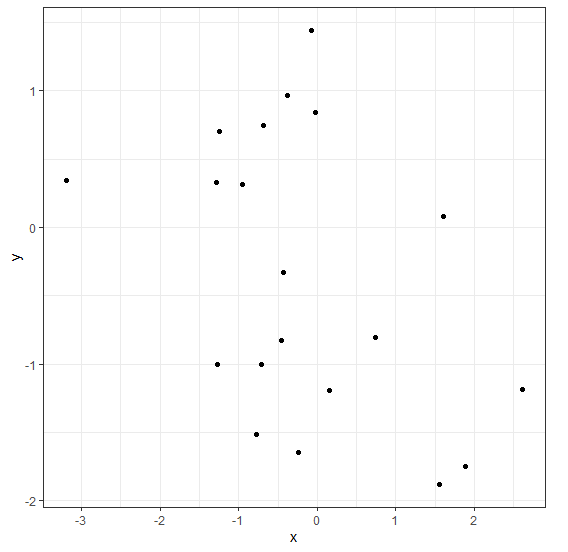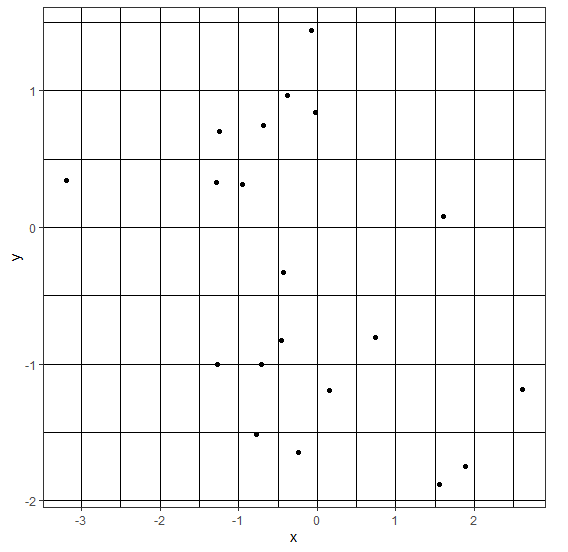# Create darker gridlines in theme_bw for a ggplot2 graph in R.

To create darker gridlines in theme_bw for a ggplot2 graph, we can use theme function, where we can use major and minor gridlines element line to black color with the help of panel.grid.major and panel.grid.minor argument as shown in the below Example.

We can use any other color but black is the most preferred one as it matches with the border color.

## Example

Following snippet creates a sample data frame −

x<-rnorm(20)
y<-rnorm(20)
df<-data.frame(x,y)
df

The following dataframe is created

         x           y
1 -0.68202228  0.74932718
2 -1.27191457 -1.00033680
3 -0.38075101  0.96859452
4  0.73811131 -0.80209795
5 -0.45029624 -0.82566426
6  1.60531737  0.07919983
7 -0.02205589  0.84328336
8  1.55123302 -1.88301265
9 -3.19483717   0.34585608
10 1.89040486  -1.74882186
11 -0.71422305 -1.00218331
12 -1.28397783  0.33301330
13  2.61596121 -1.18867702
14 -0.95879700  0.31557380
15  0.15064137 -1.19404095
16 -0.24493887 -1.64769752
17 -0.78022098 -1.51595008
18 -1.24295882  0.70125826
19 -0.07898175  1.44606626
20 -0.42474934 -0.32754527

To load ggplot2 package and create scatterplot between x and y in black and white theme on the above created data frame, add the following code to the above snippet −

x<-rnorm(20)
y<-rnorm(20)
df<-data.frame(x,y)
library(ggplot2)
ggplot(df,aes(x,y))+geom_point()+theme_bw()

## Output

If you execute all the above given snippets as a single program, it generates the following Output −To create scatterplot between x and y in black and white theme with darker gridlines on the above created data frame, add the following code to the above snippet −

x<-rnorm(20)
y<-rnorm(20)
df<-data.frame(x,y)
library(ggplot2)
ggplot(df,aes(x,y))+geom_point()+theme_bw()+theme(panel.grid.major=element_line
(colour="black"))+theme(panel.grid.minor=element_line(colour="black"))

## Output

If you execute all the above given snippets as a single program, it generates the following Output −